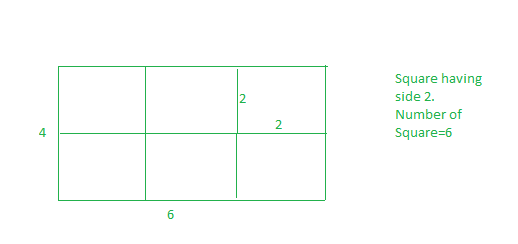GFG App
Open AppBrowser
Continue

# Minimum squares to evenly cut a rectangle

Given a rectangular sheet of length l and width w. we need to divide this sheet into square sheets such that the number of square sheets should be as minimum as possible.
Examples:

Input :l= 4 w=6
Output :6
We can form squares with side of 1 unit, But the number of squares will be 24, this is not minimum. If we make square with side of 2, then we have 6 squares. and this is our required answer.
And also we can’t make square with side 3, if we select 3 as square side, then whole sheet can’t be converted into squares of equal length.Input :l=3 w=5
Output :15

Optimal length of the side of a square is equal to GCD of two numbers

## C++

 `// CPP program to find minimum number of` `// squares to make a given rectangle.` `#include ` `using` `namespace` `std;`   `int` `countRectangles(``int` `l, ``int` `w)` `{` `    ``// if we take gcd(l, w), this` `    ``// will be largest possible` `    ``// side for square, hence minimum` `    ``// number of square.` `    ``int` `squareSide = __gcd(l, w);`   `    ``// Number of squares.` `    ``return` `(l * w) / (squareSide * squareSide);` `}`   `// Driver code` `int` `main()` `{` `    ``int` `l = 4, w = 6;` `    ``cout << countRectangles(l, w) << endl;` `    ``return` `0;` `}`

## Java

 `// Java program to find minimum number of` `// squares to make a given rectangle.`   `class` `GFG{` `static` `int` `__gcd(``int` `a, ``int` `b) {` `   ``if` `(b==``0``) ``return` `a;` `   ``return` `__gcd(b,a%b);` `}` `static` `int` `countRectangles(``int` `l, ``int` `w)` `{` `    ``// if we take gcd(l, w), this` `    ``// will be largest possible` `    ``// side for square, hence minimum` `    ``// number of square.` `    ``int` `squareSide = __gcd(l, w);`   `    ``// Number of squares.` `    ``return` `(l * w) / (squareSide * squareSide);` `}`   `// Driver code` `public` `static` `void` `main(String[] args)` `{` `    ``int` `l = ``4``, w = ``6``;` `    ``System.out.println(countRectangles(l, w));` `}` `}` `// This code is contributed by mits`

## Python3

 `# Python3 code to find minimum number of` `# squares to make a given rectangle.`   `import` `math `   `def` `countRectangles(l, w):`   `    ``# if we take gcd(l, w), this` `    ``# will be largest possible` `    ``# side for square, hence minimum` `    ``# number of square.` `    ``squareSide ``=` `math.gcd(l,w)` `    `  `    ``# Number of squares.` `    ``return` `(l``*``w)``/``(squareSide``*``squareSide)`   `# Driver Code` `        `  `if` `__name__ ``=``=` `'__main__'``:` `    ``l ``=` `4` `    ``w ``=` `6` `    ``ans ``=` `countRectangles(l, w)` `    ``print` `(``int``(ans))`   `# this code is contributed by` `# SURENDRA_GANGWAR`

## C#

 `// C# program to find minimum number of` `// squares to make a given rectangle.`   `class` `GFG{` `static` `int` `__gcd(``int` `a, ``int` `b) {` `if` `(b==0) ``return` `a;` `return` `__gcd(b,a%b);` `}` `static` `int` `countRectangles(``int` `l, ``int` `w)` `{` `    ``// if we take gcd(l, w), this` `    ``// will be largest possible` `    ``// side for square, hence minimum` `    ``// number of square.` `    ``int` `squareSide = __gcd(l, w);`   `    ``// Number of squares.` `    ``return` `(l * w) / (squareSide * squareSide);` `}`   `// Driver code` `public` `static` `void` `Main()` `{` `    ``int` `l = 4, w = 6;` `    ``System.Console.WriteLine(countRectangles(l, w));` `}` `}` `// This code is contributed by mits`

## PHP

 ``

## Javascript

 ``

Output:

`6`

Time Complexity: O(log(min(a, b))), where a and b are two parameters of gcd.

Auxiliary Space: O(log(min(a, b)))

My Personal Notes arrow_drop_up